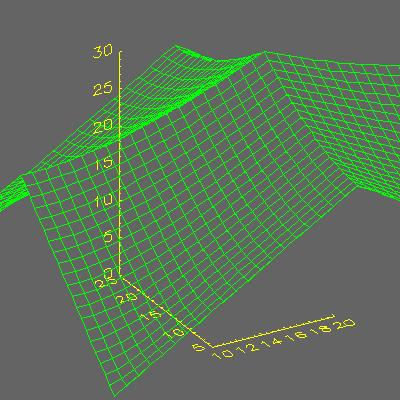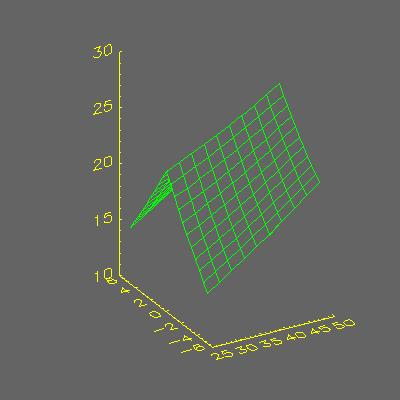# Drawing a Portion of an IDL Surface

QUESTION: I want to draw just a portion of a surface, but I find I can't use the [XY]Range keywords to do so. How can I restrict the data ranges of the surface without using these keywords?ANSWER: Yes. If you are used to plotting just a portion of a data set by using the [XY]Range keywords on a Plot command, for example, you will find it odd that you can't do the same thing with the Surface command. If you try, perhaps by typing these commands:

```   data = Dist(40, 40)
Surface, data, XRange=[10:20], YRange=[5,25]
```

the results look like this illustration:You see that the surface is not clipped, although the axes do recognize the XRange and YRange keywords, and the keywords are certainly accepted by the Surface command.

The correct way to display just a portion of the surface is to include the X and Y vectors on the Surface command and to subscript them with respect to the data range you wish to display.

For example, consider this code, in which the X vector goes from 0 to 100 and the Y vector goes from -10 to +10.

```
x = Findgen(40) * 99/39.0
y = Findgen(40) * 20/39.0 - 10.0
```

If you want to display just that portion of the data that is between 25 and 50 in X and between -5 and +5 in Y, then you might write this code:

```   Surface, data[10:19, 10:29], x[10:19], y[10:29]
```

The results are shown in this illustration. (Notice that the Y axis is auto-scaling. This can be prevented, of course, by setting the YStyle keyword to 1.)The discussion of this topic on the IDL newsgroup encouraged Martin Schultz (mgs@io.harvard.edu) of Harvard University to write a surface clipping program, named Surf_Clip. It can be used with the [XY]Range keywords, like this:

```   x = Findgen(100)
y = Findgen(50)
z = Dist(100,50)
zc = Surf_Clip(z, x, y, XRange=[20,80], YRange=[20,40], \$
XClip=xc, YClip=yc
Surface,zc,xc,yc
```[Return to IDL Programming Tips]